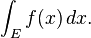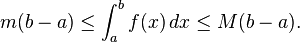# Blog Archives

## MULTIPLE INTEGRATION

Integrals can be taken over regions other than intervals. In general, an integral over a set E of a function f is written:Here x need not be a real number, but can be another suitable quantity, for instance, a vector in R3. Fubini’s theorem shows that such integrals can be rewritten as an iterated integral. In other words, the integral can be calculated by integrating one coordinate at a time.

## IMPROPER INTEGRALS

A “proper” Riemann integral assumes the integrand is defined and finite on a closed and bounded interval, bracketed by the limits of integration. An improper integral occurs when one or more of these conditions is not satisfied. In some cases such integrals may be defined by considering the limit of a sequence of proper Riemann integrals on progressively larger intervals.

If the interval is unbounded, for instance at its upper end, then the improper integral is the limit as that endpoint goes to infinity.

## INEQUALITIES FOR INTEGRALS

A number of general inequalities hold for Riemann-integrable functions defined on a closed and bounded interval [a, b] and can be generalized to other notions of integral (Lebesgue and Daniell).

• Upper and lower bounds. An integrable function f on [a, b], is necessarily bounded on that interval. Thus there are real numbers m and M so that mf (x) ≤ M for all x in [a, b]. Since the lower and upper sums of f over [a, b] are therefore bounded by, respectively, m(ba) and M(ba), it follows thatRead the rest of this entry

## LEBESGUE INTEGRAL

The Riemann integral is not defined for a wide range of functions and situations of importance in applications (and of interest in theory). For example, the Riemann integral can easily integrate density to find the mass of a steel beam, but cannot accommodate a steel ball resting on it. This motivates other definitions, under which a broader assortment of functions are integrable (Rudin 1987). The Lebesgue integral, in particular, achieves great flexibility by directing attention to the weights in the weighted sum.

The definition of the Lebesgue integral thus begins with a measure, μ. In the simplest case, the Lebesgue measure μ(A) of an interval A = [a,b] is its width, ba, so that the Lebesgue integral agrees with the (proper) Riemann integral when both exist. In more complicated cases, the sets being measured can be highly fragmented, with no continuity and no resemblance to intervals.

## RIEMANN INTEGRAL

The Riemann integral is defined in terms of Riemann sums of functions with respect to tagged partitions of an interval. Let [a,b] be a closed interval of the real line; then a tagged partition of [a,b] is a finite sequenceRiemann sums converging as intervals halve, whether sampled at ■ right, ■ minimum, ■ maximum, or ■ left.

This partitions the interval [a,b] into n sub-intervals [xi−1, xi] indexed by i, each of which is “tagged” with a distinguished point ti ∈ [xi−1, xi]. A Riemann sum of a function f with respect to such a tagged partition is defined asRead the rest of this entry

## Reviewing the regularity theory of elliptics PDEs via the Laplace equation [Part III]

Let us recall Green’s identity, if${u,v}$ are any functions smooth in${\bar{\Omega}}$ and${\Omega}$ is a bounded domain with smooth boundary we have$\displaystyle \int_\Omega u\Delta v - v \Delta u dx = \int_{\partial \Omega}u\frac{\partial v}{\partial \nu}-v\frac{\partial u}{\partial \nu}dS$

this identity can be obtained with a couple of integration by parts involving the vector fields${u \nabla v}$ and${v \nabla u}$.

Lets rewrite the identity as$\displaystyle \int_\Omega u\Delta vdx = -\int_\Omega v \Delta u dx + \int_{\partial \Omega}u\frac{\partial v}{\partial \nu}-v\frac{\partial u}{\partial \nu}dS$

thus, at least formally, if somehow we could find for every${x \in \Omega}$ a function${v_x(y)}$ such that$\displaystyle \Delta v_x(y)=\delta_x(y)$$\displaystyle v_x(y) \equiv 0 \mbox{ on } \partial \Omega$

## Reviewing the regularity theory of elliptics PDEs via the Laplace equation [Part I]

There is a tedious, simple but hopefully fruitful exercise I always wanted to do. It is to review all the different proofs of the Harnack inequality and regularity of solutions to elliptic equations that I know, but only for the Laplace equation. First, because it is a good way to really get your hands on some of the ideas of several deep theorems (like those of De Giorgi-Nash-Moser and Krylov-Safonov) in the simplest possible setting. Second, because looking at all the different proofs it is possible to trace the evolution of analysis and PDEs through the last century (and a bit before that) and appreciate the level maturity reached in several fields: potential theory, singular integrals, calculus of variations, fully non linear elliptic PDE and free boundary problems. The simple’ and elementary’ Laplace equation lies at the intersection of all these fields, so every new breakthrough reflected on our understanding of this equation, each new proof emphasizing a different approach or point of view. Each of the proofs that I will discuss are based on one of the following: